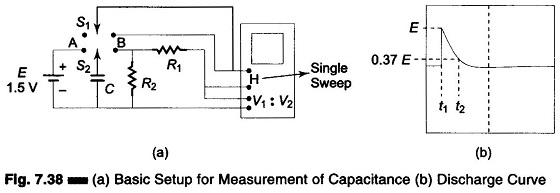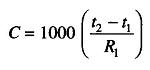## Measure of Capacitance and Inductance in Oscilloscope:

Measure of Capacitance and Inductance in Oscilloscope – Capacitance may be measured from a  capacitor discharge curve. This requires a dc oscilloscope with a triggered single sweep that is of the storage type.

Figure 7.38 (a) shows the test setup. C is the capacitor under test, and E is 1.5 V battery for charging the capacitor. R1 is the precision 1 W non-inductive resistor. (R1 should be 100 K for testing Capacitance from 10 pf – 0.1 μf, 10 K for 0.1 – 10µf, 1 K for 10 – 100 μf and 100 Ω for 100 – 1000 μf) R2 is a 1 MΩ resistor to isolate R1 from the oscilloscope input resistance.When the DPDT switch (S1 – S2) is connected to position A, capacitor C is charged by the battery; when this switch is connected to position B, the capaci­tor discharges through R1. At this time, section S1 of the switch triggers a single sweep of the oscilloscope. The shape of the discharge curve which is displayed on the screen is shown in Fig. 7.38 (b). When the (S1 – S2) switch is connected to position B, the voltage across R1 rises suddenly to the fully charged value E at time t1. The capacitor immediately begins to discharge through R1. At time t2 the voltage has fallen to 37% of the maximum. The unknown capacitor (in 1.tf) is determined from the time interval between t1 and t2 (in ms) and the resistance R1 in ohms.

ThereforeIf a single trace oscilloscope is used, the time interval t2 – t1 may be measured from (i) the calibrated sweep, and (ii) the calibrated horizontal axis.

Inductances may be measured by means of a time constant method similar to that used in the Capacitance case. Figure 7.39 (a) shows the test setup. L is the inductor under test and E is a battery or filtered dc power supply. R1 is the rheostat for adjusting the current level. A dc ammeter or mA can be temporarily inserted in series with the battery for indicating the maximum safe operating current of the L. The resistance of the rheostat at this setting must be noted.

When the DPST switch is open, no current flows through the inductor and the oscilloscope receives no signal voltage. When the switch is closed, S1 closes the dc circuit, and current flows through L and R1 in series. This produces a voltage drop across R1, which is the signal voltage applied to the oscilloscope. At the same time, S2 closes the trigger circuit and initiates a single sweep of the oscilloscope. Because of the counter emf generated by the inductor, the current increases exponentially, as shown in Fig. 7.39 (b). At time t1, the voltage has risen to 63% of its final or maximum value, E. The unknown inductance (in Henries) is determined from the time interval between t0 and t1 (t0 = 0) and resistance R1 • t1 and t0 may be measured (i) from the calibrated sweep and (ii) from the time calibrated horizontal axis.

Scroll to Top